# Math in Focus Grade 4 Chapter 12 Practice 4 Answer Key Composite Figures

This handy Math in Focus Grade 4 Workbook Answer Key Chapter 12 Practice 4 Composite Figures detailed solutions for the textbook questions.

## Math in Focus Grade 4 Chapter 12 Practice 4 Answer Key Composite Figures

Find the lengths of the unknown sides of each figure. Then find the perimeter of each figure.

Example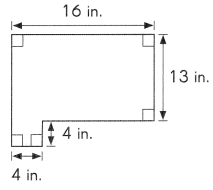Length of first unknown side = 16 – 4 = 12 in.
Length of second unknown side = 13 + 4 = 17 in.
Perimeter of figure = 16 + 13 + 12 + 4 + 4 + 17 = 66 in.
Perimeter = 66 in.

Question 1.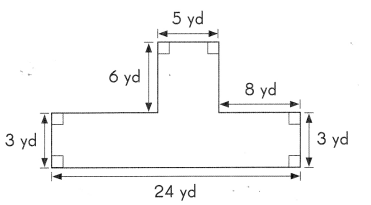Perimeter = ____________ yd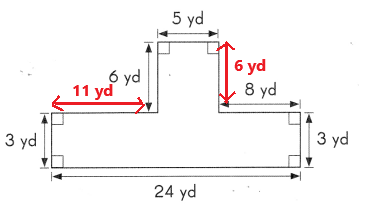Length of first unknown side = 6 yards
Length of second unknown side = 24 yards – 8 yards – 5 yards = 11 yards
Perimeter of figure = 5 yd + 6 yd + 8 yd + 3 yd + 24 yd + 3 yd + 11 yd +6 yd
= 66 yd
Perimeter = 66 yd.

Question 2.
Tom wants to fence in the piece of land shown in the diagram. Find the perimeter of the piece of land to find the length of fencing material he needs.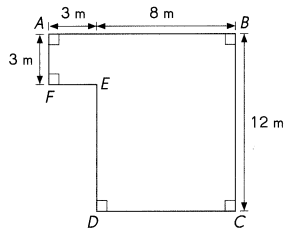Perimeter = __________ m
Length of first unknown side = 8 m
Length of second unknown side = 9 m
Length of third unknown side = 3m
Perimeter of the piece of land = 3 m + 8 m + 12 m + 8 m + 9 m + 3 m + 3 m = 46 m
Perimeter = 46 m
He needs 46 m of fencing material.

Question 3.
Find the perimeter of this figure.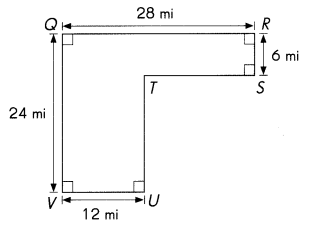Perimeter = ___________ mi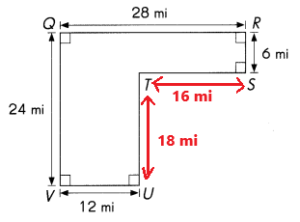Length of first unknown side ST = 28 mi – 12 mi = 16 mi
Length of second unknown side TU = 24 mi – 6 mi = 18 mi
Perimeter of the figure = 28 mi + 6 mi + 16 mi + 18 mi + 12 mi + 24 mi = 104 mi
Perimeter = 104 mi

Question 4.
Find the perimeter of the figure.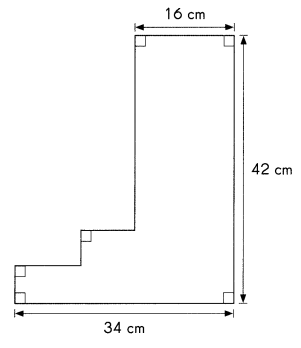Perimeter = ___________ cm
Perimeter  of the figure = 42 cm + 18 cm + 34 cm + 42 cm + 16 cm = 152 cm
Perimeter = 152  cm

Find the area of each composite figure. Show your work.

Example

Break up the figure into two rectangles as shown. Then find the area of the whole figure.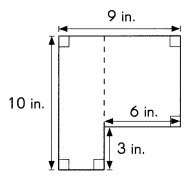Area of rectangle 1 = length × width
= 10 × 3
= 30 in.2
Area of rectangle 2 = length × width
= 7 × 6
= 42 in.2
Total area = area of rectangle 1 + area of rectangle 2
= 30 + 42
= 72 in.2
Area = 72 in.2

Find the area of each composite figure. Show your work.

Question 5.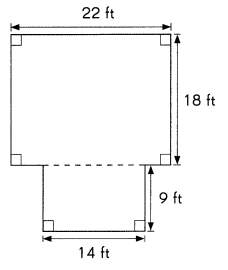Area = ____________ ft2
For rectangle 1
length = 22 feet
Width = 18 feet
Area of rectangle 1 = length × width
= 22 feet × 18 feet
= 396 ft2
For rectangle 2
length = 14 feet
width = 9 feet
Area of rectangle 2 = length × width
= 14 feet × 9 feet
= 126 ft2
Total area = area of rectangle 1 + area of rectangle 2
= 396 ft2+ 126 ft2
= 522 ft2
Area =522 ft2

Question 6.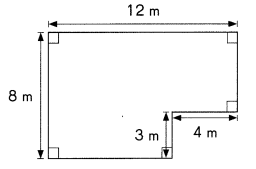Area = __________ m2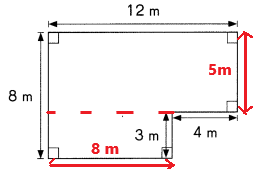Explanation:
For rectangle 1
length = 12 m
Width = 5 m
Area of rectangle 1 = length × width
= 12 m × 5 m
= 60 m2
For rectangle 2
length = 8 m
width = 3 m
Area of rectangle 2 = length × width
= 8 m × 3 m
= 24 m2
Total area = area of rectangle 1 + area of rectangle 2
= 60 m2+ 24 m2
=84 m2
Area =84 m2